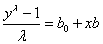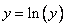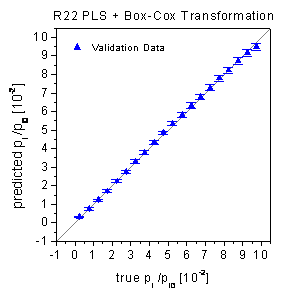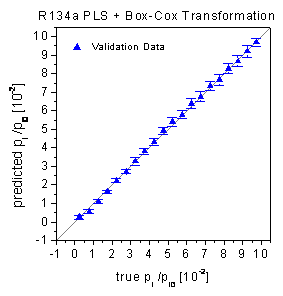Home News About Me Ph. D. Thesis Abstract Table of Contents 1. Introduction 2. Theory  Fundamentals of the Multivariate Data Analysis 3. Theory  Quantification of the Refrigerants R22 and R134a: Part I 4. Experiments, Setups and Data Sets 5. Results  Kinetic Measurements 6. Results  Multivariate Calibrations 6.1. PLS Calibration 6.2. Box-Cox Transformation + PLS 6.3. INLR 6.4. QPLS 6.5. CART 6.6. Model Trees 6.7. MARS 6.8. Neural Networks 6.9. PCA-NN 6.10. Neural Networks and Pruning 6.11. Conclusions 7. Results  Genetic Algorithm Framework 8. Results  Growing Neural Network Framework 9. Results  All Data Sets 10. Results  Various Aspects of the Frameworks and Measurements 11. Summary and Outlook 12. References 13. Acknowledgements Publications Research Tutorials Downloads and Links Contact Search Site Map Print this Page## 6.2.   Box-Cox Transformation + PLS

The Box-Cox transformation or power transformation is a general and widely used linearization procedure when no theory exists, which indicates that a certain transformation of the input and/or response variables will result in a more linear model . The idea is to model a power of the response variable y as a linear function of x:(27)

The value of l, which fits the linear function of x best, is estimated using the available data of the pure analytes. After the estimation of l and of the regression coefficients b and b0, the response variable can be transformed according to:(28)

If l=0, it is common to transform y according to:(29)

was determined for the measurements of the single refrigerants of the calibration data set. For R22 l=0.68 and for R134a l=0.74 were estimated. Then the relative saturation pressures of the refrigerants of the calibration and the validation data were transformed according to expression (28) . Similar to section 6.1 PLS models were built for the transformed calibration data and then the validation data were predicted whereby the optimal number of principal components was determined by the minimum error of crossvalidation of the calibration data. The optimal model for R22 contained 11 principal components and the model for R134a used 10 principal components. The calibration data were predicted with a relative RMSE of 2.97% for R22 and 4.50% for R134a. The prediction of the validation data, which is also shown in figure 34 was performed with rel. RMSE of 3.09% for R22 and 5.04% for R134a. Both, the Durbin-Watson Statistics and the Wald-Wolfowitz Runs test are significant at the 5% error level. In figure 34, it is visible that the prediction of both analytes shows slightly a wave. Compared with the standard PLS the Box-Cox Transformation allows a highly improved calibration while a few nonlinearities remain uncalibrated. Although a rather high number of principal components are needed, only a slight overfitting can be observed, as the errors of the validation data are only moderately higher than the errors of the calibration data.figure 34:  True-predicted plots of the PLS for the validation data. The data were linearized by a Box-Cox transformation.

 Page 91 © Frank Dieterle, 03.03.2019